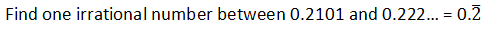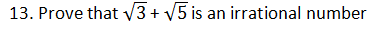Chapter 1 Number System R.D. Sharma Solutions for Class 9th Math Exercise 1.4
x
Exercise 1.4

1. Define an irrational number .

Solution

An irrational number is a real number that cannot be reduced to any ratio between an integer p and a natural number q. If the decimal representation of an irrational number is non-terminating and non-repeating, then it is called irrational number. For example, √3 = 1.732....

2. Explain, how irrational numbers differ from rational numbers ?

Solution

Every rational number must have either terminating or non-terminating but irrational number must have non- terminating and non-repeating decimal representation.  A rational number is a number that can be written as simple fraction (ratio) and denominator is not equal to zero while an irrational is a number that cannot be written as a ratio.

3. Examine, whether the following numbers are rational or irrational:Solution

(i) Let x = √7
Therefore,
x = 2.645751311064...

It is non-terminating and non-repeating
Hence, √7 is an irrational number

(ii) Let x = √4
Therefore,
x = 2
It is terminating.
Hence √4 is a rational number.

(iii) Let x = 2 +√3 be the rational
Squaring on both sides,
⇒ x2 = (2+√3)2
⇒ x2 = 4+3+4√3
⇒ x2 = 7+4√3
⇒ x2-7 = 4√3
⇒ (x2-7)/4 = √3
Since, x is rational
⇒ x2 is rational.
⇒ x2-7 is rational
⇒ (x2-7)/4 is rational
⇒ √3  is rational
But, √3 is irrational
So, we arrive at a contradiction.
Hence 2+√3 is an irrational number.

(iv) Let x = √3+√2 be the rational number
Squaring on both sides, we get
⇒ x2 = (√3+√2)2
⇒ x2 = 3+2+2√6
⇒ x2 = 5+2√6
⇒ x2-5 = 2√6
⇒ (x2-5)/2 = √6
Since, x is a rational number
⇒ x2 is rational number
⇒ x2 -5 is rational number
⇒ (x2-5)/2 is rational number
⇒ √6 is rational number
But √6 is an irrational number
Hence, √3+√2 is an irrational number

(v) Let x = √3+√5 be the rational number
Squaring on both sides, we get
⇒ x2 = (√3+√5)2
⇒ x2 = 3+5+2√15
⇒ x2 = 8+2√15
⇒ x2 -8 = 2√15
⇒ (x2 -8)/2 = √15
Now, x is rational number
⇒ x2 is rational number
⇒ x2-8 is rational number
(x2 -8)/2 is rational number
⇒ √15 is rational number.
But √15 is an irrational number
So, we arrive at a contradiction
Hence, √3+√5 is an irrational number

(vi) Let x = (√2-2)2 be a rational number.
x = (√2-2)2
⇒ x = 2+4-4√2
⇒ x = 6-4√2
⇒ x-6/-4 = √2
Since, x is rational number,
⇒ x – 6 is a rational nummber
⇒ x-6/-4 is a rational number
⇒ √2 is a rational number
But we know that √2 is an irrational number, which is a contradiction
So, (√2-2)2 is an irrational number

(vii) Let x = (2-√2) (2+√2)
⇒ x = 22 - √2 {as (a+b) (a-b) = 22 - 22}
⇒ x = 4-2
⇒ x = 2
So, (2-√2) (2+√2) is a rational number

(viii) Let x = (√2+√3)2 be rational number
Using the formula, (a+b)2 = 22+ 22+2ab
⇒ x = √22+ √32+2.√2.√3
⇒ x = 2+3+2√6
⇒ x = 5+2√6
⇒ x-5/2 = √6
⇒ x-5/2 is a rational number
⇒ √6 is a rational number
But we know that √6 is an irrational number
So, we arrive at a contradiction
So, (√2+√3)2 is an irrational number.(x) Let
x = √23
⇒ x = 4.79583...
It is non-terminating or non-repeating
Hence, √23 is an irrational number

(xi) Let x = √225
⇒ x = 15
Hence √225 is a rational number

(xii) Given 0.3796
It is terminating
Hence, it is a rational number

(xiii) Given number x = 7.478478
⇒ x = 71428
It is repeating
Hence, it is a rational number

(xiv) Given number is x = 1.1010010001...
It is non-terminating or non-repeating
Hence, it is an irrational number

4. Identify the following as rational or irrational numbers. Give the decimal representation of rational numbers:Solution
(i) Given number is x = √4
x = 2, which is a rational number(iii) Given number is √1.44
Now, we have to check whether it is rational or irrational.
⇒ √1.44 = √(144/100)
⇒ √1.44 = √144/100
⇒ √1.44 = 12/10
⇒ √1.44 = 6/5
⇒ √1.44 = 1.2
So, it is a rational

(iv) Given that √(9/27) = √9/27
Now, we have to check whether it is rational or irrational
√(9/27) = √9/27
⇒ √(9/27) = 3/3√3
⇒ √(9/27) = 1/√3
So, it is an irrational number

(v) Given that √-64
Now, we have to check whether it is rational or irrational
Since, -√64 = -8
So, it is a rational number

(vi) Given that √100
Now, we have to check whether it is rational or irrational
Since, √100 = 10
So, it is rational number

5. In the following equations, find which variables x, y, z etc. represent rational or irrational numbers:
(i) x2 = 5
(ii)  y2 = 9
(iii)  z2 = 0.004
(iv)  u2 = 17/4
(v)  v2 = 3
(vi)  w2 = 27
(vii)  t2 = 0.4

Solution

(i) Given that x2 = 5
Now, we have to find the value of x.
Since x2 = 5
⇒ x = √5
So, it x is an irrational number

(ii) Given that y2 = 9
Now, we have to find the value of y.
y2 = 9
⇒ y = √9
⇒ y = 3
So, y is a rational number

(iii) Given that z2 = 0.04
Now, we have to find the value of z.
⇒ z2 = 4/100
⇒ z = √(4/100)
⇒ z = 2/10
⇒ z = 1/5
So, it is rational number

(iv) Given that u2 = 17/4
Now, we have to find the value of u.
u2 = 17/4
⇒ u = √(17/4)
⇒ u = √(17/2)
So, it is an irrational number

(v) Given that, v2 = 3
Now, we have to find the value of v
v2 = 3
⇒ v = √3
So, it is an irrational number

(vi) Given that, w2 = 27
Now, we have to find the value of w.
⇒ w = √27
w = 3√3
So, it is an irrational number

(vii) Given that t2 = 0.4
Now, we have to find the value of t
⇒ t = √0.4
⇒ t = √(4/10)
⇒ t = 2/√10
So, it is an irrational number

6. Give an example of each, of two irrational numbers whose:
(i) difference is a rational number.
(ii) difference is an irrational number.
(iii) sum is a rational number.
(iv) sum is an irrational number.
(v) product is an rational number.
(vi) product is an irrational number.
(vii) quotient is a rational number.
(viii) quotient is an irrational number.

Solution

(i) Let √2, 1+√2
So, 1+√2 - √2 = 1
Therefore, √2 and 1+√2 are two irrational numbers and their difference is a rational number.

(ii) Let 4√3, 3√3 are two irrational numbers and their difference is an irrational number.
Because 4√3- 3√3 = √3 is an irrational number.

(iii) Let √5, -√5 are two irrational numbers and their sum is a rational number.
That is √5+(-√5) = 0.

(iv) Let 2√5, 3√5 are two irrational numbers and their sum is an irrational number.
That is 2√5 + 3√5 = 5√5

(v) Let √8, √2 are two irrational numbers and their product is a rational number.
That is √8×√2 = √16 = 4

(vi) Let √2, √3 are two irrational numbers and their product is an irrational number
That is √2×√3 = √6

(vii) Let √8, √2 are two irrational numbers and their quotient is a rational number
That is √8/√2 = 2√2/√2 = 2

(viii) Let √2, √3 are two irrational numbers and their quotient is an irrational number
That is √2፥√3 = √2/√3

7. Give two rational numbers lying between 0.232332333233332... and 0.212112111211112.

Solution

Let,
a = 0.232332333233332…..
b = 0.212112111211112…..
Here, the decimal representation of a and b are non-terminating and non-repeating. So we observe that in first decimal place of a and b have the same digit 2 but digit in the second place of their decimal representation are distinct. And the number a has 3 and b has 1. So a > b.
Hence, two rational numbers are 0.222 lying between 0.232332333233332….. and 0.212112111211112…..

8. Give two rational numbers lying between 0.515115111511115...0.5353353335...

Solution

Let a = 0.515115111511115… and b = 0.535335333533335….

Here, the decimal representation of a and b are non-terminating and non-repeating. So we observe that in first decimal place a and b have the same digit 5 but digit in the second place of their decimal representation are distinct. And the number a has 1 and b has 3. So a < b.

Hence, two rational numbers are 0.5152,0.532 lying between 0.515115111511115….and 0.535335333533335….

9.Solution

Let,
a = 0.2101
b = 0.2222….
Here, a and are rational numbers .Since a has terminating and b has repeating decimal. We observe that in second decimal place a has 1 and b has 2. So a < b.
Hence one irrational number is 0.220100100010000…. lying between 0.2101 and 0.2222….

10. Find a rational number and also an irrational number lying between the numbers 0.3030030003 ... and 0.3010010001 ...

Solution

Let,
a = 0.3030030003…
b = 0.3010010001….
Here, decimal representation of a and b are non-terminating and non-repeating. So a and b are irrational numbers. We observe that in first two decimal place of a and b have the same digit but digit in the third place of their decimal representation is distinct. Therefore, a > b.
Hence one rational number is 0.3011 lying between 0.3030030003….. and 0.3010010001… and irrational number is 0.3020200200020000… lying between 0.3030030003….and 0.3010010001….

11. Find two irrational numbers between 0.5 and 0.55.

Solution

Let ,
a = 0.5
b = 0.55
Here, a and b are rational number. So we observe that in first decimal place a and b have same digitSo a < b. Hence two irrational numbers are 0.510100100010000… and 0.5202002000200002… lying between 0.5 and 0.55.

12. Find two irrational numbers lying between 0.1 and 0.12.

Solution

Let,
a = 0.1
b = 0.12
Here, and b are rational number. So we observe that in first decimal place a and b have same digit. So a < b.
Hence, two irrational numbers are 0.1010010001… and 0.11010010001…lying between 0.1 and 0.12.Solution

Given that √3+√5 is an irrational number
Now, we have to prove √3+√5 is an irrational number
Let x = √3+√5 is a rational.
Squaring on both sides,
x2 = (√3 + √5)2
⇒ x2 = (√3)2 + (√5)2 + 2√3×√5
⇒ x2 = 3+5+2√15
⇒ (x2 = 8 + 2√15
⇒ (x2-8)/2 = √15
Now, x is rational
⇒ x2 is rational
⇒ (x2-8)/2 is rational
⇒ √15 is rational
But, √15 is an irrational
Thus, we arrive at contradiction that √3+√5 is a rational which is wrong.
Hence, √3+√5 is an irrationalSolution

Let x = 5/7 = 0.714285 and y = 9/11 = 0.81

Here, we observe that in the first decimal x has digit 7 and y has 8. So x < y. In the second decimal place x has digit 1. So, if we considering irrational numbers
a = 0.72072007200072..
b = 0.73073007300073..
c = 0.74074007400074....
We find that,
x<a<b<c<y
Hence, a,b,c are required irrational numbers.